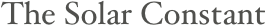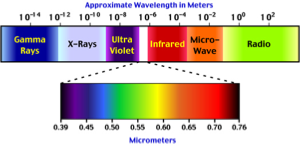Scientists look for the easy way out.  This concept is a fine example.  We assume that the sun is a constant source of energy--that solar output of radiation is constant.  Why do we do this? Is there any evidence for it?

According to Wikipedia:

The solar constant, a measure of flux, is the amount of incoming solar electromagnetic radiation per unit area that would be incident on a plane perpendicular to the rays, at a distance of one astronomical unit (AU) (roughly the mean distance from the Sun to the Earth). When solar irradiance is measured on the outer surface of Earth's atmosphere, the measurements can be adjusted using the inverse square law to infer the magnitude of solar irradiance at one AU and deduce the solar constant.

The solar constant includes all types of solar radiation, not just the visible light. It is measured by satellite to be roughly 1.366 kilowatts per square meter (kW/m²). The actual direct solar irradiance at the top of the atmosphere fluctuates by about 6.9% during a year (from 1.412 kW/m² in early January to 1.321 kW/m² in early July) due to the Earth's varying distance from the Sun, and typically by much less than one part per thousand from day to day. Thus, for the whole Earth (which has a cross section of 127,400,000 km²), the power is 1.740×1017 W, plus or minus 3.5%. The solar constant does not remain constant over long periods of time (see Solar variation), but over a year varies much less than the variation of direct solar irradiance at the top of the atmosphere arising from the ellipticity of the Earth's orbit. The approximate average value cited, 1.366 kW/m², is equivalent to 1.96 calories per minute per square centimeter, or 1.96 langleys (Ly) per minute.

Is the sun providing the same energy to the earth every day, or is it varying?

How can we know this?

Radiation from the sun---93 million miles in 8.3 minutes (186,000 miles/s)